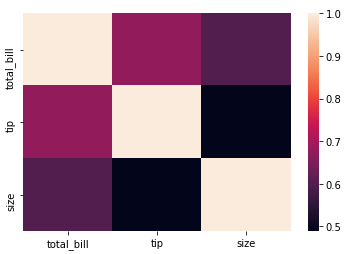# How to create heat maps in Python?

In this post, let’s see how to create heat maps in Python.

## Heat Maps

A heat map is a graphical, two-dimensional representation of data. It uses different color shades to differentiate values.

Example

For example, a dark green in a heat map may represent a higher value, whereas a light green may represent a lower value. The vice-versa may also apply.

## Suitable applications of heat maps

Heat maps are best suited for matrix data. Such as correlation data or a confusion matrix. Such matrices are predominant in the field of data science.

Correlation matrix between total bill and tips at a restaurant

total_bill tip size
total_bill 1.000000 0.675734 0.598315
tip 0.675734 1.000000 0.489299
size 0.598315 0.489299 1.000000

## How to create heat maps in Python

In Python, a heatmap can be created using the Seaborn library, provided you have the data at hand.

Let’s say, I have the above correlation matrix as a Pandas dataframe under the name data.

```data
```
total_bill tip size
total_bill 1.000000 0.675734 0.598315
tip 0.675734 1.000000 0.489299
size 0.598315 0.489299 1.000000

To create the heatmap, import the Seaborn library as “sns”.

```import seaborn as sns
```

Our next step is to use the below code to straightaway generate the heatmap.

```sns.heatmap(data)
```## Customizing heat maps with attributes

### annot

Let’s add the values on each cell of the above heatmap. Just turn on the annot attribute to True.

```sns.heatmap(data, annot= True)
```### cbar

Now, since the values are available on the heat map itself, there is no need for the color sidebar. Hence, we can remove them by adding the cbar attribute.

```sns.heatmap(data, annot= True, cbar = False)
```### cmap

Also, you can change the color map of the above heat map as well from the default colors. Use cmap attribute.

```sns.heatmap(data, annot= True, cbar = False,
cmap = "YlGnBu")
```In this way, we can create heat maps in python and also customize as per our needs.

Use Shift+Tab while your cursor is at the “sns.heatmap” code to explore all the attributes of this function. With those attributes, you can further fine-tune and customize the heatmaps.

## One thought to “How to create heat maps in Python?”

1.wow says:

Spr